# Perimeter, Area and Volume - Stage 3

These resources are designed to encourage you to explore perimeter, area and volume of shapes and solids based on rectangles and triangles. For resources about area, perimeter and volume that include shapes and solids with curved edges and surfaces, see our collection Perimeter, Area and Volume - Stage 4.

Scroll down to see the complete collection, or explore our subcollections on Perimeter and Area in two dimensions, and Surface Area and Volume in three dimensions.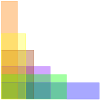### Perimeter and Area

This selection of problems is a great starting point for learning about Perimeter and Area.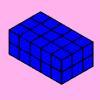### Surface Area and Volume

This selection of problems is a great starting point for learning about Surface Area and Volume### Blue and White

##### Age 11 to 14Challenge Level

Identical squares of side one unit contain some circles shaded blue. In which of the four examples is the shaded area greatest?### An Unusual Shape

##### Age 11 to 14Challenge Level

Can you maximise the area available to a grazing goat?### Cuboids

##### Age 11 to 14Challenge Level

Can you find a cuboid that has a surface area of exactly 100 square units. Is there more than one? Can you find them all?### On the Edge

##### Age 11 to 14Challenge Level

If you move the tiles around, can you make squares with different coloured edges?### Sending a Parcel

##### Age 11 to 14Challenge Level

What is the greatest volume you can get for a rectangular (cuboid) parcel if the maximum combined length and girth are 2 metres?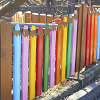### Fence It

##### Age 11 to 14Challenge Level

If you have only 40 metres of fencing available, what is the maximum area of land you can fence off?### Efficient Cutting

##### Age 11 to 14Challenge Level

Use a single sheet of A4 paper and make a cylinder having the greatest possible volume. The cylinder must be closed off by a circle at each end.### Isosceles Triangles

##### Age 11 to 14Challenge Level

Draw some isosceles triangles with an area of $9$cm$^2$ and a vertex at (20,20). If all the vertices must have whole number coordinates, how many is it possible to draw?### Cola Can

##### Age 11 to 14Challenge Level

An aluminium can contains 330 ml of cola. If the can's diameter is 6 cm what is the can's height?### Can They Be Equal?

##### Age 11 to 14Challenge Level

Can you find rectangles where the value of the area is the same as the value of the perimeter?### Cuboid Challenge

##### Age 11 to 16Challenge Level

What's the largest volume of box you can make from a square of paper?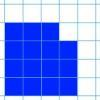### Changing Areas, Changing Perimeters

##### Age 11 to 14Challenge Level

How can you change the area of a shape but keep its perimeter the same? How can you change the perimeter but keep the area the same?### Changing Areas, Changing Volumes

##### Age 11 to 14Challenge Level

How can you change the surface area of a cuboid but keep its volume the same? How can you change the volume but keep the surface area the same?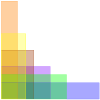### Perimeter Possibilities

##### Age 11 to 14Challenge Level

I'm thinking of a rectangle with an area of 24. What could its perimeter be?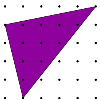### Triangles in a Square

##### Age 11 to 14Challenge Level

What are the possible areas of triangles drawn in a square?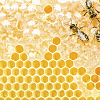### Perimeter Challenge

##### Age 11 to 14Challenge Level

Can you deduce the perimeters of the shapes from the information given?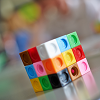### Colourful Cube

##### Age 11 to 14Challenge Level

A colourful cube is made from little red and yellow cubes. But can you work out how many of each?### Isometric Areas

##### Age 11 to 14Challenge Level

We usually use squares to measure area, but what if we use triangles instead?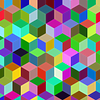### More Isometric Areas

##### Age 11 to 14Challenge Level

Isometric Areas explored areas of parallelograms in triangular units. Here we explore areas of triangles...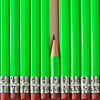### Perimeter, Area and Volume Short Problems

##### Age 11 to 16

A collection of short problems on area and volume.### Shear Magic

##### Age 11 to 14Challenge Level

Explore the area of families of parallelograms and triangles. Can you find rules to work out the areas?### Triangle in a Trapezium

##### Age 11 to 16Challenge Level

Can you find and prove the relationship between the area of a trapezium and the area of a triangle constructed within it?